ICSE Class 9 Mid-point and Its Converse Solution New Pattern

## ICSE Class 9 Mid-point and Its Converse Solution New Pattern By Clarify Knowledge

ICSE Class 9 Mid-point and Its Converse Solution New Pattern 2022

## Chapter 12 - Mid-point and Its Converse [ Including Intercept Theorem] Exercise Ex. 12(A)

Question 1

In triangle ABC, M is mid-point of AB and a straight line through M and parallel to BC cuts AC in N. Find the lengths of AN and MN if Bc = 7 cm and Ac = 5 cm.Solution 1

The triangle is shown below,

Since M is the midpoint of AB and MN||BC hence N is the midpoint of AC.Therefore

And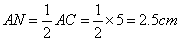Question 2

Prove that the figure obtained by joining the mid-points of the adjacent sides of a rectangle is a rhombus.Solution 2

The figure is shown below,

Let ABCD be a rectangle where P,Q,R,S are the midpoint of AB,BC, CD, DA.We need to show that PQRS is a rhombus

For help we draw two diagonal BD and AC as shown in figure

Where BD=AC(Since diagonal of rectangle are equal)

Proof:

From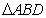and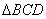Similarly 2PQ=2SR=AC and PQ||SR----- (2)

From (1) and (2) we get

PQ=QR=RS=PS

Therefore PQRS is a rhombus.

Hence provedQuestion 3

D, E and F are the mid-points of the sides AB, BC and CA of an isoscelesABC in which AB = BC. Prove thatDEF is also isosceles.Solution 3

The figure is shown below

Given that ABC is an isosceles triangle where AB=AC.

Since D,E,F are midpoint of AB,BC,CA therefore

2DE=AC and 2EF=AB this means DE=EF

Therefore DEF is an isosceles triangle an DE=EF.

Hence provedQuestion 4

The following figure shows atrapezium ABCD in which AB // DC. P is the mid-point of AD and PR // AB. Prove that:

Solution 4

Here from triangle ABD P is the midpoint of AD and PR||AB, therefore Q is the midpoint of BD

Similarly R is the midpoint of BC as PR||CD||AB

From triangle ABD 2PQ=AB …….(1)

From triangle BCD 2QR=CD …..(2)

Now (1)+(2)=>

2(PQ+QR)=AB+CD

Hence provedQuestion 5

The figure, given below, shows a trapezium ABCD. M and N are the mid-point of the non-parallel sides AD and BC respectively. Find:

(i) MN, if AB = 11 cm and DC = 8 cm.

(ii) AB, if Dc = 20 cm and MN = 27 cm.

(iii) DC, if MN = 15 cm and AB = 23 cm.Solution 5

Let we draw a diagonal AC as shown in the figure below,

(i)Given that AB=11cm,CD=8cm

From triangle ABC

From triangle ACD

Hence MN=OM+ON=(4+5.5)=9.5cm

(ii)Given that CD=20cm,MN=27cm

From triangle ACD

Therefore ON=27-10=17cm

From triangle ABC

(iii)Given that AB=23cm,MN=15cm

From triangle ABC

Therefore OM=15-11.5=3.5cm

From triangle ACD

Question 6

The diagonals of a quadrilateral intersect at right angles. Prove that the figure obtained by joining the mid-points of the adjacent sides of the quadrilateral is rectangle.Solution 6

The figure is shown below

Let ABCD be a quadrilateral where P,Q,R,S are the midpoint of AB,BC,CD,DA. Diagonal AC and BD intersects at right angle at point O. We need to show that PQRS is a rectangle

Proof:

Fromand2PQ=AC and PQ||AC …..(1)

2RS=AC and RS||AC …..(2)

From (1) and (2) we get,

PQ=RS and PQ||RS

Similarly we can show that PS=RQ and PS||RQ

Therefore PQRS is a parallelogram.

Now PQ||AC, thereforeAgain BD||RQ, therefore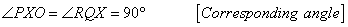Similarly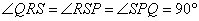Therefore PQRS is a rectangle.

Hence provedQuestion 7

L and M are the mid-point of sides AB and DC respectively of parallelogram ABCD. Prove that segments DL and BM trisect diagonal AC.Solution 7

The required figure is shown below

From figure,

BL=DM and BL||DM and BLMD is a parallelogram, therefore BM||DL

From triangle ABY

L is the midpoint of AB and XL||BY, therefore x is the midpoint of AY.ie AX=XY …..(1)

Similarly for triangle CDX

CY=XY …..(2)

From (1) and (2)

AX=XY=CY and AC=AX+XY+CY

Hence provedQuestion 8

ABCD is a quadrilateral in which AD = BC. E, F, G and H are the mid-points of AB, BD, CD and Ac respectively. Prove that EFGH is a rhombus.

Solution 8

From the figure,

For triangle ADC and triangle ABD

For triangle BCD and triangle ABC

2GF=BC and 2EH=BC, therefore 2GF=2EH=BC …..(3)

From (1),(2),(3) we get,

2GH=2EF=2GF=2EH

GH=EF=GF=EH

Therefore EFGH is a rhombus.

Hence provedQuestion 9

A parallelogram ABCD has P the mid-point of Dc and Q a point of Ac such that CQ =AC. PQ produced meets BC at R.

Prove that

(i)R is the midpoint of BC

(ii)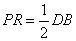Solution 9

For help we draw the diagonal BD as shown below

The diagonal AC and BD cuts at point X.

We know that the diagonal of a parallelogram intersects equally each other. Therefore

AX=CX and BX=DX

Given,

Therefore Q is the midpoint of CX.

(i)For triangle CDX PQ||DX or PR||BD

Since for triangle CBX

Q is the midpoint of CX and QR||BX. Therefore R is the midpoint of BC

(ii)For triangle BCD

As P and R are the midpoint of CD and BC ,thereforeQuestion 10

D, E and F are the mid-points of the sides AB, BC and CA respectively ofABC. AE meets DF at O. P and Q are the mid-points of OB and OC respectively. Prove that DPQF is a parallelogram.Solution 10

The required figure is shown below

For triangle ABC and OBC

2DE=BC and 2PQ=BC, therefore DE=PQ …..(1)

For triangle ABO and ACO

2PD=AO and 2FQ=AO, therefore PD=FQ …..(2)

From (1),(2) we get that PQFD is a parallelogram.

Hence provedQuestion 11

In triangle ABC, P is the mid-point of side BC. A line through P and parallel to CA meets AB at point Q; and a line through Q and parallel to BC meets median AP at point R. Prove that

(i)AP=2AR

(ii)BC=4QRSolution 11

The required figure is shown below

From the figure it is seen that P is the midpoint of BC and PQ||AC and QR||BC

Therefore Q is the midpoint of AB and R is the midpoint of AP

(i)Therefore AP=2AR

(ii)Here we increase QR so that it cuts AC at S as shown in the figure.

(iii)From triangle PQR and triangle ARS

Therefore QR=RS

Now

Hence provedQuestion 12

In trapezium ABCD, AB is parallel to DC; P and Q are the mid-points of AD and BC respectively. BP produced meets CD produced at point E. Prove that:

(i) Point P bisects BE,

(ii) PQ is parallel to AB.Solution 12

The required figure is shown below

(i)

(ii)For tiangle ECB PQ||CE

Again CE||AB

Therefore PQ||AB

Hence provedQuestion 13

In a triangle ABC, AD is a median and E is mid-point of median AD. A line through B and E meets AC at point F.

Prove that: AC = 3AFSolution 13

The required figure is shown below

For help we draw a line DG||BF

Now from triangle ADG, DG||BF and E is the midpoint of AD

Therefore F is the midpoint of AG,ie AF=GF …..(1)

From triangle BCF, DG||BF and D is the midpoint of BC

Therefore G is the midpoint of CF,ie GF=CF …(2)

AC=AF+GF+CF

AC=3AF(From (1) and (2))

Hence provedQuestion 14

D and F are mid-points of sides AB and AC of a triangle ABC. A line through F and parallel to AB meets BC at point E.

(i) Prove that BDFE is parallelogram

(ii) Find AB, if EF = 4.8 cm.Solution 14

The required figure is shown below

(i)Since F is the midpoint and EF||AB.

Therefore E is the midpoint of BC

So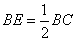and…..(1)

Since D and F are the midpoint of AB and AC

Therefore DE||BC

SO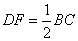and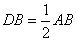…..(2)

From (1),(2) we get

BE=DF and BD=EF

Hence BDEF is a parallelogram.

(ii)Since

Question 15

In triangle ABC, AD is the median and DE, drawn parallel to side BA, meets AC at point E. Show that BE is also a median.Solution 15

Question 16

In ∆ABC, E is mid-point of the median AD and BE produced meets side AC at point Q. Show that BE : EQ = 3:1.Solution 16

Question 17

In the given figure, M is mid-point of AB and DE, whereas N is mid-point of BC and DF. Show that: EF = AC.

Solution 17

## Chapter 12 - Mid-point and Its Converse [ Including Intercept Theorem] Exercise Ex. 12(B)

Question 1

Use the following figure to find:

(i) BC, if AB = 7.2 cm.

(ii) GE, if FE = 4 cm.

(iii) AE, if BD = 4.1 cm

(iv) DF, if CG = 11 cm.

Solution 1

According to equal intercept theorem since CD=DE

Therefore AB=BC and EF=GF

(i)BC=AB=7.2cm

(ii)GE=EF+GF=2EF=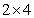=8cm

Since B,D,F are the midpoint and AE||BF||CG

Therefore AE=2BD and CG=2DF

(iii)AE=2BD=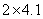=8.2

(iv)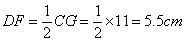Question 2

In the figure, give below, 2AD = AB, P is mid-point of AB, Q is mid-point of DR and PR // BS. Prove that:

(i) AQ // BS

(ii) DS = 3 Rs.

Solution 2

Given that AD=AP=PB as 2AD=AB and p is the midpoint of AB

(i)From triangle DPR, A and Q are the midpoint of DP and DR.

Therefore AQ||PR

Since PR||BS ,hence AQ||BS

(ii)From triangle ABC, P is the midpoint and PR||BS

Therefore R is the midpoint of BC

DS=DQ+QR+RS=QR+QR+RS=3RSQuestion 3

The side AC of a triangle ABC is produced to point E so that CE =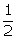AC. D is the mid-point of BC and ED produced meets AB at F. Lines through D and C are drawn parallel to AB which meet AC at point P and EF at point R respectively. Prove that:

(i) 3DF = EF(ii) 4CR = AB.Solution 3

Consider the figure:

Here D is the midpoint of BC and DP is parallel to AB, therefore P is the midpoint of AC and(i)

Again from the triangle AEF we have AE ||PD||CR andThereforeor we can say that 3DF = EF .

Hence it is shown.

(ii)

From the triangle PED we have PD||CR and C is the midpoint of PE thereforeNow

Hence it is shown. Question 4

In triangle ABC, the medians BP and CQ are produced upto points M and N respectively such that BP = PM and CQ = QN. Prove that:

(i) M, A and N are collinear.

(ii) A is the mid-point of MN.Solution 4

The figure is shown below

(i)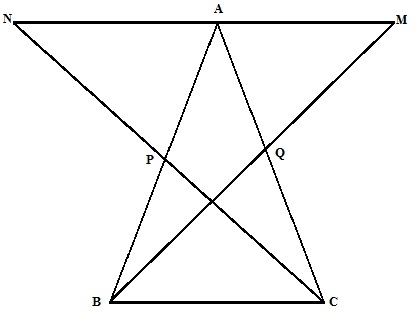From triangle BPC and triangle APN

And BC=AN ……(3)

Similarly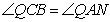…..(2)

And BC=AM ……(4)

Now

Therefore M,A,N are collinear

(ii) From (3) and (4) MA=NA

Hence A is the midpoint of MNQuestion 5

In triangle ABC, angle B is obtuse. D and E are mid-points of sides AB and BC respectively and F is a point on side AC such that EF is parallel to AB. Show that BEFD is a parallelogram.Solution 5

The figure is shown below

From the figure EF||AB and E is the midpoint of BC.

Therefore F is the midpoint of AC.

Here EF||BD, EF=BD as D is the midpoint of AB

BE||DF, BE=DF as E is the midpoint of BC.

Therefore BEFD is a parallelogram.Question 6

In parallelogram ABCD, E and F are mid-points of the sides AB and CD respectively. The line segments AF and BF meet the line segments ED and EC at points G and H respectively. Prove that:

(i) Triangles HEB and FHC are congruent;

(ii) GEHF is a parallelogram.Solution 6

The figure is shown below

(i)

(ii)

Similarly AG=GF and EG=DG …..(1)

For triangle ECD, F and H are the midpoint of CD and EC.

Therefore HF||DE and…..(2)

(1),(2) we get, HF=EG and HF||EG

Similarly we can show that EH=GF and EH||GF

Therefore GEHF is a parallelogram.Question 7

In triangle ABC, D and E are points on side AB such that AD = DE = EB. Through D and E, lines are drawn parallel to BC which meet side AC at points F and G respectively. Through F and G, lines are drawn parallel to AB which meet side BC at points M and N respectively. Prove that: BM = MN = NC.Solution 7

The figure is shown below

For triangle AEG

D is the midpoint of AE and DF||EG||BC

Therefore F is the midpoint of AG.

AF=GF …..(1)

Again DF||EG||BC DE=BE, therefore GF=GC …..(2)

(1),(2) we get AF=GF=GC.

Similarly Since GN||FM||AB and AF=GF ,therefore BM=MN=NC

Hence provedQuestion 8

In triangle ABC; M is mid-point of AB, N is mid-point of AC and D is any point in base BC. Use intercept Theorem to show that MN bisects AD.Solution 8

The figure is shown below

Since M and N are the midpoint of AB and AC, MN||BC

According to intercept theorem Since MN||BC and AM=BM,

Therefore AX=DX. Hence provedQuestion 9

If the quadrilateral formed by joining the mid-points of the adjacent sides of quadrilateral ABCD is a rectangle, show that the diagonals AC and BD intersect at right angle.Solution 9

The figure is shown below

Let ABCD be a quadrilateral where P,Q,R,S are the midpoint of AB,BC,CD,DA.PQRS is a rectangle. Diagonal AC and BD intersect at point O. We need to show that AC and BD intersect at right angle.

Proof:

PQ||AC, therefore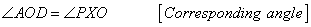….(1)

||Again BD||RQ, therefore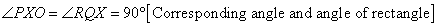…(2)

From (1) and (2) we get ,

SimilarlyTherefore diagonals AC and BD intersect at right angle

Hence provedQuestion 10

In triangle ABC; D and E are mid-points of the sides AB and AC respectively. Through E, a straight line is drawn parallel to AB to meet BC at F. Prove that BDEF is a parallelogram. If AB = 16 cm, AC = 12 cm and BC = 18 cm, find the perimeter of the parallelogram BDEF.Solution 10

The figure is shown below

From figure since E is the midpoint of AC and EF||AB

Therefore F is the midpoint of BC and 2DE=BC or DE=BF

Again D and E are midpoint ,therefore DE||BF and EF=BD

Hence BDEF is a parallelogram.

Now

Therefore perimeter of BDEF=2(BF+EF)=Question 11

In the given figure, AD and CE are medians and DF//CE. Prove that:

error: Content is protected !!